Test: Power Systems- 6

# Test: Power Systems- 6 - Electrical Engineering (EE)

Test Description

## 10 Questions MCQ Test GATE Electrical Engineering (EE) 2023 Mock Test Series - Test: Power Systems- 6

Test: Power Systems- 6 for Electrical Engineering (EE) 2023 is part of GATE Electrical Engineering (EE) 2023 Mock Test Series preparation. The Test: Power Systems- 6 questions and answers have been prepared according to the Electrical Engineering (EE) exam syllabus.The Test: Power Systems- 6 MCQs are made for Electrical Engineering (EE) 2023 Exam. Find important definitions, questions, notes, meanings, examples, exercises, MCQs and online tests for Test: Power Systems- 6 below.
Solutions of Test: Power Systems- 6 questions in English are available as part of our GATE Electrical Engineering (EE) 2023 Mock Test Series for Electrical Engineering (EE) & Test: Power Systems- 6 solutions in Hindi for GATE Electrical Engineering (EE) 2023 Mock Test Series course. Download more important topics, notes, lectures and mock test series for Electrical Engineering (EE) Exam by signing up for free. Attempt Test: Power Systems- 6 | 10 questions in 30 minutes | Mock test for Electrical Engineering (EE) preparation | Free important questions MCQ to study GATE Electrical Engineering (EE) 2023 Mock Test Series for Electrical Engineering (EE) Exam | Download free PDF with solutions
 1 Crore+ students have signed up on EduRev. Have you?
Test: Power Systems- 6 - Question 1

### A three phase transmission line having a self-reactance of 1 Ω/km and a mutual reactance of 0.2 Ω/km. The negative and zero sequence equivalent reactances respectively are

Detailed Solution for Test: Power Systems- 6 - Question 1

Positive sequence reactance = Xs – Xm = 0.8 Ω/km

Negative sequence reactance = Xs – Xm = 0.8 Ω/km

Zero sequence reactance = Xs + 2Xm = 1.4 Ω/km

Test: Power Systems- 6 - Question 2

### A balanced star connected load takes 50 A from a balanced 3-phase. 4-wire supply. The zero and positive sequence components (Iao and Ia1) of the line current (Ia) are respectively

Detailed Solution for Test: Power Systems- 6 - Question 2

The given load is balanced star connected load. In balanced system only positive sequence component is present, both negative and zero sequence components are absent.

Zero sequence component, Iao = 0 A

Given that, Ia = 50A

Positive sequence component,

Ia1 = Ia = 50A

Test: Power Systems- 6 - Question 3

### What is the per unit reactance of an alternator of the capacity is doubled and the voltage is halved?

Detailed Solution for Test: Power Systems- 6 - Question 3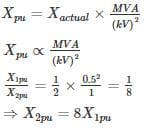*Answer can only contain numeric values
Test: Power Systems- 6 - Question 4

A transmission line has an impedance of 0.3 PU on 11 KV and 200 MVA base. Find the new PU Impedance on 33 kV and 1600 MVA base

Detailed Solution for Test: Power Systems- 6 - Question 4

ZPUnew = 0.3 ×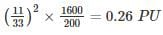Test: Power Systems- 6 - Question 5

A transmission line of inductance 0.1 H and resistance 5 ohms is suddenly short-circuited at t = 0 at the bar end ass shown in the figure. The approximate value of the first current maximum (Maximum momentary current) is

Detailed Solution for Test: Power Systems- 6 - Question 5

Z = 5 + j 314 × 0.1 = 5 + j 31.4 = 31.8 ∠81°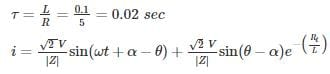= 3.14 sin (314 t - 66) + 2.87 e-50 t

First current maximum of symmetrical short circuit current occurs at

57.3 × 314 t - 66 = 90°

⇒ t = 0.00867 sec

First current maximum

imm = 3.14 + 2.87 e-50 × 0.00867 = 5 A

*Answer can only contain numeric values
Test: Power Systems- 6 - Question 6

Two power stations S1 and S2 are interconnected through a transmission line of per unit (p.u) reactance of 0.6. Station S1 has one generator of P.U. the reactance of 0.3, and station S2 has two generators of p.u reactance 0.6 each. For a 3 – phase symmetrical short circuit at the middle of the transmission line (point F). The change in the value of the equivalent fault reactance, with one of the generators of S2 removed, is _____ (in %).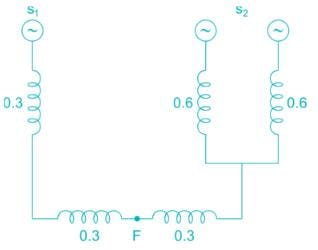Detailed Solution for Test: Power Systems- 6 - Question 6

Equivalent reactance with respect to fault point =(0.3 + 0.3) || (0.3 + (0.6 || 0.6))

=0.6 || (0.3 + 0.3)

= 0.3

After removal of one of the generators of S2 with respect to fault point

= (0.3 + 0.3) || (0.3 + 0.6)

= (0.6) || (0.9) = 0.36
Change in the reactance =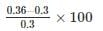= 20%

*Answer can only contain numeric values
Test: Power Systems- 6 - Question 7

For a generator, the ratio of fault current for Line to Line fault and three phase fault is 0.5.

The positive sequence reactance is 0.2 μ. The negative sequence reactance is __(in p.u.)

Detailed Solution for Test: Power Systems- 6 - Question 7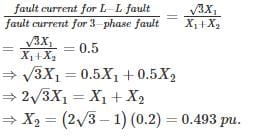Test: Power Systems- 6 - Question 8

A three-phase Y-Δ transformer is rated 400 MVA, 220 kV/22 kV. The short circuit impedance of the transformer measured on low voltage side is 0.121 Ω and because of the low value, it is considered equal to the leakage reactance. The per unit value of reactance to represent this transformer in a system whose base on the high voltage side of the transformer is 100 MVA, 230 kV is ___ (pu)

Detailed Solution for Test: Power Systems- 6 - Question 8

On its own base the pu reactance of the transformer is,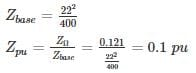On the chosen base the reactance becomes,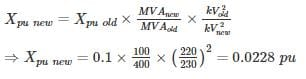*Answer can only contain numeric values
Test: Power Systems- 6 - Question 9

A 3ϕ load is connected to a 3ϕ balanced supply Van = 20∠120°, Vbn = 20∠-120°, Vcn = 20∠0° find the value of R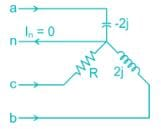Detailed Solution for Test: Power Systems- 6 - Question 9

Apply KCL
Ia + Ib + Ic = In = 0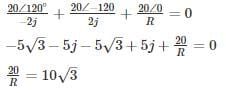R = 1.154 Ω

Test: Power Systems- 6 - Question 10

Two 300 MVA, 22 kV, 3-phase, 60 Hz alternators are connected by a transmission line having transformers at both ends as shown in the single line diagram given below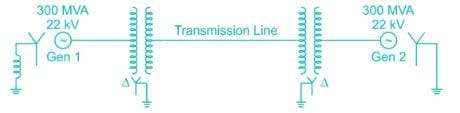The transformer (T1) between the generator 1 and the transmission line is a Δ-Y connected 3-phase transformer bank made up of three single phase transformers each rated at 120 MVA, 22 kV/127 kV with leakage reactance of 8%. The generator 2 is connected to the line by a 3-phase, 300 MVA, 22 kV/220 kV, Δ-Y transformer (T2) with 10% leakage reactance. The star side of T1 and T2 are grounded. The zero sequence reactance of the transmission line is 81 Ω. Considering the MVA rating of T1 as the base, the zero-sequence network of the complete system is (Assume Xg1 and Xg2 are the zero sequence reactance of the generators respectively)

Detailed Solution for Test: Power Systems- 6 - Question 10

Given that base MVA is the rating of T1

Rating of single phase transformer of 3-phase transformer bank = 12 MVA

Rating of 3-phase transformer bank = 3 × 120 = 360 MVA

It is connected in Δ Y connection.

Primary voltage of three phase transformer bank = Primary voltage of single phase transformer = 22 kV

Secondary voltage of three phase transformer bank = √3 × secondary voltage of single phase transformer = √3 × 127kV = 220kV

XT1 (for single phase transformer) = j0.08

XT1 = (for three phase transformer)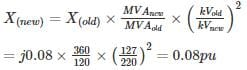XT1 = j0.08 pu

XT2 (old) = j0.10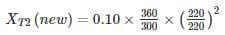= j 0.12 pu

Given that, reactance of transmission line (XL) = 81Ω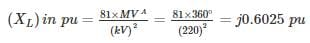As the generator reactance values are not given, let assume zero sequence reactance of generator 1 and generator 2 are Xg1 and Xg2 respectively.

Now, the zero-sequence network becomes.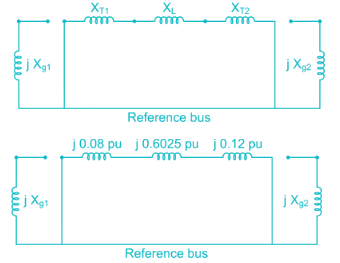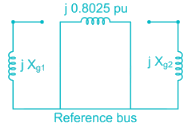## GATE Electrical Engineering (EE) 2023 Mock Test Series

22 docs|284 tests
Information about Test: Power Systems- 6 Page
In this test you can find the Exam questions for Test: Power Systems- 6 solved & explained in the simplest way possible. Besides giving Questions and answers for Test: Power Systems- 6, EduRev gives you an ample number of Online tests for practice

## GATE Electrical Engineering (EE) 2023 Mock Test Series

22 docs|284 tests(Scan QR code)